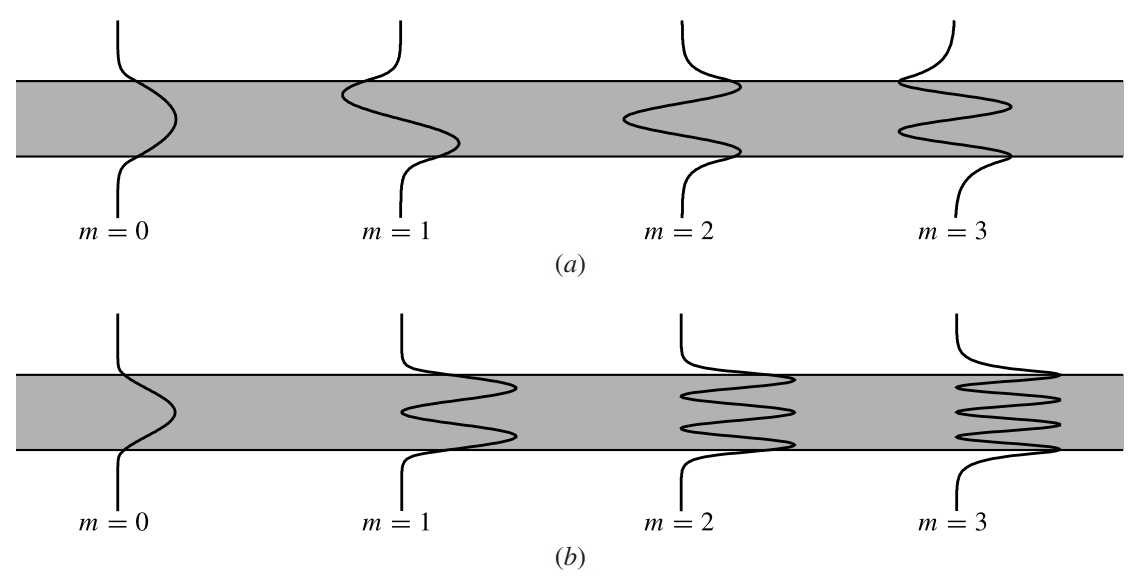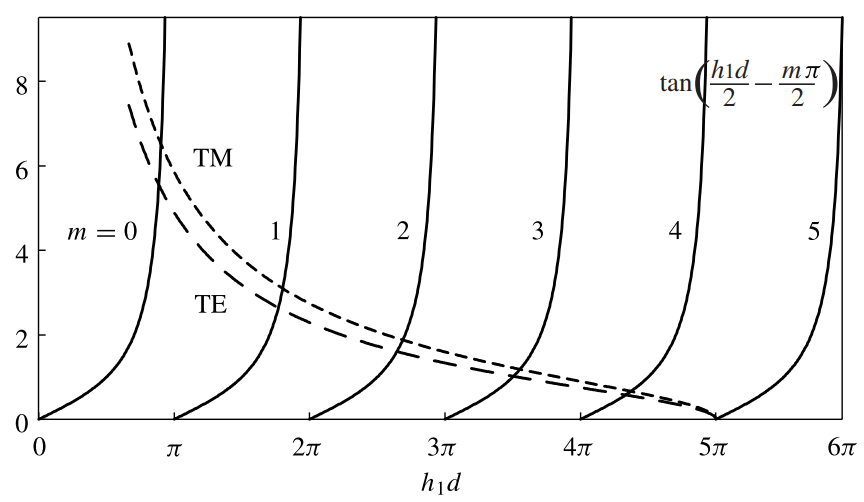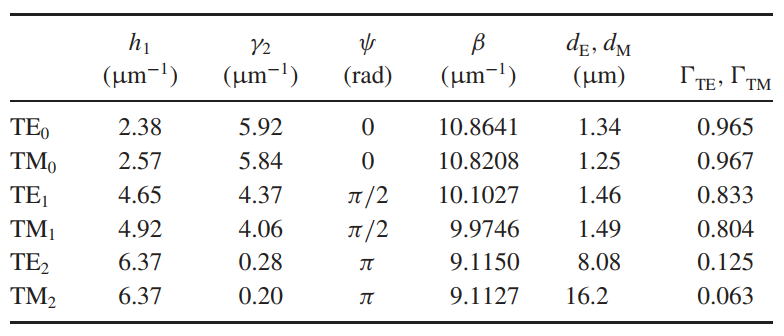# Symmetric Slab Waveguides

This is a continuation from the previous tutorial - Step-Index Planar Waveguides.

In a symmetric slab waveguide, n3 = n2 and aE = aM = 0. In addition, we also have γ3 = γ2. Then, it can be seen from (57) and (62) [refer to the step-index planar waveguides tutorial] that $$\tan2\psi=0$$ and

$\tag{83}\psi=\frac{m\pi}{2}, \qquad m = 0, 1, 2, ...,$

for both TE and TM modes. Therefore, the mode field patterns of a symmetric waveguide given by (55) and (60) are either even functions of x with cos(h1x) in the region -d/2 < x < d/2 for even values of m or odd functions of x with sin(h1x) in the region -d/2 < x < d/2 for odd values of m. This characteristic is expected because the mode field pattern in a symmetric structure is either symmetric or antisymmetric. Figure 9 below shows the field patterns and the corresponding intensity distributions of the first few guided modes of a symmetric slab waveguide.Figure 9   (a) Field patterns and (b) intensity distributions of the first few guided modes of a symmetric slab waveguide.

By equating γ3 to γ2 and using the following identity

$\tag{84}\tan2\theta=\frac{2\tan\theta}{1-\tan^2\theta}=\frac{2\cot\theta}{\cot^2\theta-1},$

the eigenvalue equation (56) [refer to the step-index planar waveguides tutorial] for TE modes can be transformed to the following two equations:

$\tag{85}\tan\frac{h_1d}{2}=\frac{\gamma_2}{h_1},\qquad \text{for even modes},$

$\tag{86}-\cot\frac{h_1d}{2}=\frac{\gamma_2}{h_1},\qquad \text{for odd modes},$

which yield the allowed parameters of guided TE modes. These two equations can be combined in one eigenvalue equation for all guided TE modes:

$\tag{87}\tan\left(\frac{h_1d}{2}-\frac{m\pi}{2}\right)=\frac{\gamma_2}{h_1}=\frac{\sqrt{V^2-h_1^2d^2}}{h_1d},\qquad m=0,1,2,...,$

where m is the same mode number as the one in (83). Using (61) [refer to the step-index planar waveguides tutorial], a similar procedure yields

$\tag{88}\tan\left(\frac{h_1d}{2}-\frac{m\pi}{2}\right)=\frac{n_1^2}{n_2^2}\frac{\gamma_2}{h_1}=\frac{n_1^2}{n_2^2}\frac{\sqrt{V^2-h_1^2d^2}}{h_1d},\qquad m=0,1,2,...,$

for guided TM modes. The solutions of (87) yield the allowed values of h1d for a given value of the waveguide parameter V for both even and odd TE modes. Those of (88) yield the allowed values of h1d for both even and odd TM modes. Figure 10 below shows an example with V = 5π. Because n1 > n2, it can be seen from comparison of (87) and (88) and from the graphic solution shown in figure 10 that for modes of the same order, $$h_1^{TE} < h_1^{TM}$$. This is consistent with the conclusion obtained from the general discussions in the step-index planar waveguides tutorial.

Because aE = aM = 0, TE and TM modes of a symmetric waveguide have the same cutoff condition:

$\tag{89}V_m^c=m\pi$

for the mth TE and TM modes alike. This can also be seen in figure 10 below.Figure 10  Graphic solutions for the eigenvalues of guided TE and TM modes of a symmetric waveguide of V = 5π. The intersections of the dashed and solid curves yield the values of h1d for eigenmodes.

Because m = 0 for the fundamental modes, neither fundamental TE nor fundamental TM mode in a symmetric waveguide has cutoff. Any symmetric dielectric waveguide supports at least one TE and one TM mode. The number of TE modes supported by a given symmetric waveguide is the same as that of the TM modes and is simply

$\tag{90}M_{TE}=M_{TM}=\left[\frac{V}{\pi}\right]_\text{int}.$

These conclusions are unique to symmetric waveguides. They are not true for an asymmetric waveguide. For example, a guided mode for an asymmetric slab waveguide at a given optical frequency may not exist because both its fundamental TE and TM modes have a nonzero cutoff.

The confinement factors of the modes of a symmetric waveguide have the following simple forms:

$\tag{91}\Gamma_{TE}=\frac{\gamma_2d(V^2+2\gamma_2d)}{V^2(2+\gamma_2d)}$

and

$\tag{92}\Gamma_{TM}=\frac{\gamma_2d(q_2V^2+2\gamma_2d)}{V^2(2+q_2\gamma_2d)}$

In general, the confinement factor has to be calculated by first solving the eigenvalue equation of a particular mode to find the mode parameters and then by using (91) or (92), or, in the case of an asymmetric waveguide, (79) and (80) [refer to the step-index planar waveguides tutorial]. However, for the fundamental TE mode of a symmetric slab waveguide, the following approximate formula can be used:

$\tag{93}\Gamma_0^{TE}=\frac{V^2}{2+V^2}$

Note that (93) is a function of V only. Thus, it can be used to calculate the confinement factor of the fundamental TE mode without knowing the mode parameters. It has an error of less than 1.5%.

Example

A symmetric slab waveguide, as shown in figure 11 below, is made by sandwiching a polymer layer of thickness d = 1 µm between the silica substrate and cover as shown. At 1 µm optical wavelength, n1 = 1.77 for the polymer guiding layer, and n2 = 1.45 for the silica substrate and cover. How many guided TE and TM modes are supported by this waveguide? Find the propagation constants and the confinement factors for the guided TE and TM modes of this waveguide.

With the given parameters of the waveguide, we have V = 6.378, k1 = 11.12 µm-1, and k2 = 9.11 µm-1. Using (90), we find that the waveguide now supports three TE modes and three TM modes because

$M_{TE}=M_{TM}=\left[\frac{6.378}{\pi}\right]_\text{int}=[2.03]_\text{int}=3$

Compared with the asymmetric waveguide example in the step-index planar waveguides tutorial, two additional modes, TE2 and TM2, are supported by this symmetric waveguide. Because V/π = 2.03 is very close to the integer 2, however, these two modes are only slightly above cutoff.

The mode characteristics of this symmetric waveguide can be found in a procedure similar to, but much simpler than, that used to find those of the asymmetric waveguide in the step-index planar waveguides tutorial. By taking ξ = h1d, we see that the eigenvalue equations (87) and (88) are already expressed in terms of the variable ξ. They can be solved numerically to find the values of h1 for the guided TE and TM modes. Then, $$\beta=(k_1^2-h_1^2)^{1/2}$$ and $$\gamma_2=(\beta^2-k_2^2)^{1/2}$$ are found. The phase shift $$\psi$$ need not be solved because it is simply $$\psi=m\pi/2$$ for the mth mode. By setting γ3 = γ2 for the symmetric waveguide, the effective waveguide thickness can be calculated from (59) for a TE mode and from (64) for a TM mode. The confinement factors are found using (91) and (92) for TE and TM modes, respectively. The numerical characteristics of the guided modes of this symmetric waveguide are summarized below.We still see the same characteristics as those in the first example in the step-index planar waveguides tutorial that a TE mode has a larger propagation constant than a TM mode of the same order and that the TE0 mode has the largest propagation constant. The characteristics discussed in the second example in that tutorial for the confinement factors are also seen here for the symmetric waveguide.

Compared with the asymmetric waveguide in the first example in the step-index planar waveguides tutorial, it is interesting to see that the symmetric waveguide now supports TE2 and TM2 modes because of the increased index in the cover layer from 1, of the air, to 1.45, of silica. Because these two modes are very close to the cutoff point, they have very small values of γ2. As a consequence, their fields penetrate deeply into the cover and substrate, resulting in their large effective thickness and small confinement factors. Meanwhile, their propagation constants are only slightly larger than k2 of the substrate and cover. The field and intensity distributions of these modes have the characteristics of the symmetric waveguide modes shown in figure 9 above.

We can use (93) to obtain an estimate of $$\Gamma_0^{TE}\approx0.953$$ for V = 6.378. Compared with the actual confinement factor of 0.965 for the TE0 mode listed above, the error of (93) is only 1.2% in this case.

The next part continues with the Graded-Index Planar Waveguide tutorial.